# AP Chemistry Practice Test 4

### Test Information14 questions21 minutes

`Questions 1-4 refer to the following information.2SO2(g) + O2(g) → 2SO3(g)4.0 mol of gaseous SO2 and 6.0 mol of O2 gas are allowed to react in a sealed container.`

1. Which particulate drawing best represents the contents of the flask after the reaction goes to completion?

2. What percentage of the original pressure will the final pressure in the container be equal to?

3. At a given point in the reaction, all three gases are present at the same temperature. Which gas molecules will have the highest velocity and why?

4. Under which of the following conditions would the gases in the container most deviate from ideal conditions and why?

5. Four different acids are added to beakers of water, and the following diagrams represent the species present in each solution at equilibrium. Which acid has the highest pH?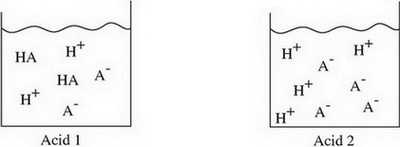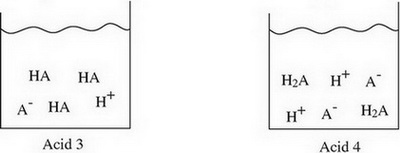6. Which expression below should be used to calculate the mass of copper that can be plated out of a 1.0 M Cu(NO3)2 solution using a current of 0.75 A for 5.0 minutes?

7. Lewis diagrams for the nitrate and nitrite ions are shown below. Choose the statement that correctly describes the relationship between the two ions in terms of bond length and bond energy.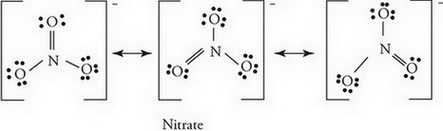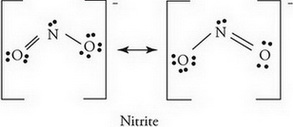8. Examining data obtained from mass spectrometry supports which of the following?

9. A 2.0 L flask holds 0.40 g of helium gas. If the helium is evacuated into a larger container while the temperature is held constant, what will the effect on the entropy of the helium be?

`Questions 10-14 refer to the following information.NO2 gas is placed in a sealed, evacuated container and allowed to decompose via the following equation:2NO2(g) ↔ 2NO(g) + O2(g)The graph below indicates the change in concentration for each species over time.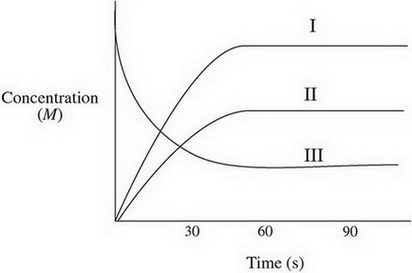`

10. Using the numbers of the lines on the graph, identify which line belongs to which species.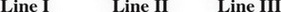11. What is happening to the rate of the forward reaction at t = 60 s?

12. As the reaction progresses, what happens to the value of the equilibrium constant Kp if the temperature remains constant?

13. What would happen to the slope of the NO2 line if additional O2 were injected into the container?

14. Using the graph, how could you determine the instantaneous rate of disappearance of NO2 at t = 30 s?